# Black Sea Scientific Journal Of Academic Research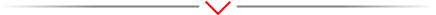Research Article - (2022) Volume 59, Issue 1

### EXPERIMENTAL STUDIES OF THE INFLUENCE OF HYDRODYNAMICALLY ACTIVE ADDITIVES ON THE OPERATION OF A SCREW COMPRESSOR

Amirov Fikret* and Amirov Elnur Gizi*

Department of Technical Sciences, Azerbaijan State University of Oil and Industry, Baku, Azerbaijan

*Correspondence: Amirov Fikret, Department of Technical Sciences, Azerbaijan State University of Oil and Industry, Baku, Azerbaijan, Email: Amirov Elnur Gizi, Department of Technical Sciences, Azerbaijan State University of Oil and Industry, Baku, Azerbaijan, Email:

Received: Mar 21, 2022, Manuscript No. BSSJAR-22-57917; Editor assigned: Mar 23, 2022, Pre QC No. BSSJAR-22-57917; Reviewed: Apr 04, 2022, QC No. BSSJAR-22-57917; Revised: Apr 06, 2022, Manuscript No. BSSJAR-22-57917; Published: Apr 13, 2022, DOI: 10.36962/GBSSJAR/59.1.001

## Abstract

Experimental study of the 79VKG-25/5 screw compressor was carried out in compressor mode on industrial oil with the addition of Polyisobutylene (PIB) at the following solution concentrations: 0.2%, 0.4%, and 0.6%. The productivity of a screw compressor practically remains constant in the range of changes in PIB concentrations from 0 to 0.2% and decreases on average by 10%. The decrease in performance is due to an increase in internal gas flows in the screw compressor. From the presented dependences it follows that in the entire range, the introduction of PIB additives does not lead to a significant increase in the air temperature at the outlet of the screw compressor and, consequently, in the pressure in the working cavity of the compressor. Therefore, the main reason for the increase in leakage and a decrease in productivity, apparently, is the deterioration of the sealing properties of the working fluid in the gaps between the housing and the rotors. The results of experimental studies are presented in the form of graphical dependences of the consumed power, volumetric productivity and isothermal efficiency of the 7VKG-25/5 screw compressor on the degree of pressure increase and polyisobutylene concentrations - in the range for the working fluid flow rates V(M1) 100 l/min and V(M2) 140 l/min.

## Keywords

Screw compressor, Centrifugal pump, Polymer additives, Polyisobutylene, Hydrodynamic losses, Fluid flow rate.

## Introduction

Experimental study of the 79VKG-25/5 screw compressor was carried out in compressor mode on industrial oil with the addition of Polyisobutylene (PIB) at the following solution concentrations: 0.2%, 0.4%, and 0.6%. At working fluid temperatures of ~45°C, at a suction air temprature of 20+27°C, at a suction pressure P_BC=1.04 kg/cm2 and an oil consumption V_M=100 and 140 l/min.

Before testing PIB solutions, the characteristics were taken on industrial oil, conventionally, the concentration of the solution K=0 is considered.

The influence of polymer additives on the characteristics of a screw compressor was determined by comparing the characteristics when operating on industrial oil and oil solutions with the addition of PIB.

## Materials and Methods

Experimental technique and results of processing

Based on these measurements, the following parameters were calculated:

1. Compressor suction pressure

a) When measured with a vacuum meter P_BC= Pδ K-0.01 n/kg/cm2/,

Where Pδ -barometric pressure; n is the number of divisions of the vacuum meter; K=0.00136 kgf/sms/mm Hgconversion factor.

b) When measuring with a U-shaped tube filled with dibutyl phtolate P_BC=0.0769 Δh _1/mm Hg/, where Δh _1 is the liquid drop in the U-tube, 0.0769 is a conversion factor.

2. Mass flow rate of the compressor G=0.0125 20 ^2 (n.g)Δh )/kg/h/, where Δh is the liquid level difference in the differential pressure gauge/mm Hg; α-coefficient of consumption; ɛ-correction factor for the expansion of the measured medium; d_20-diameter of the aperture disk hole/mm/; p_(n.g)=(P_(n.g) 10^4)/(R T_(n.g))-density pressure and, temperature of the working gas before diaphragm; P_(n.g) and T_(n.g)-respectively, the pressure and temperature of the working gas before diaphragm; R is the working gas constant.

3. Compressor volumetric capacity reduced to suction conditions: V_BC=G/(p_BC.60)/m3/min/, where p_BC=(P_BC.10^4)/(R T_BC) is the suction working gas density.

4. Power consumed by the electric motor from the network: N_el=(N_1+N_2+N_3) 1.2/kW, where N_1, N_2, N_3 are the readings of the K 505 instrument; 1.2-device coefficient.

5. Power consumed by the compressor: N_k= N_elη_el, where η_el-efficiency, electric motor, determined by its characteristics.

6. Degree of pressure rise π =P_HK/P_BC

7. Isothermal power, N_from=1/102 G R P_H/P_BC

8. Isothermal (efficiency),η_from=N_from/N_k

9.Oil consumption supplied to the working cavity of the compressor when measuring with a diaphragm: V_M=0.0125 h/), where d_20 is the diameter of the diaphragm disc opening; α-consumption coefficient; Δh -readings of the diaphragm differential pressure gauge; p is the density of the oil.

## Results

Graphs of dependences of power consumption, volumetric capacity and isothermal efficiency of the 79VKG-25/5 screw compressor on the degree of pressure increase and polyisobutylene concentrations-N_k, V, η_from the flow rates of the working fluid V_(M_1)=100 l/min and V_(M_2)=140 l/min. From their consideration, it follows that the power consumed by the compressor weakly depends on the PIB concentration in the oil. In the range of k=0-0.4% for all values ofπ , there is a tendency for N_k to decrease by 2+3%, which can be explained by a decrease in the power of hydrodynamic losses during the flow of the working fluid in the compressor. At k=0.4%, the power consumption increases by 3+5%. The results obtained are in good agreement with the data, in which the optimum concentration of PIB in industrial 45 oil was determined for a centrifugal pump 4K-6, equal to 0.35% (Figures 1-7).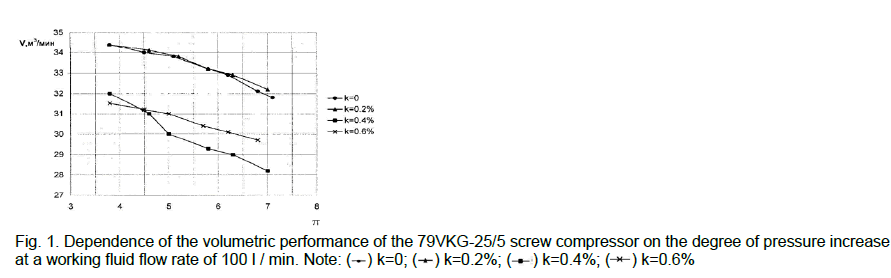Figure 1: Dependence of the volumetric performance of the 79VKG-25/5 screw compressor on the degree of pressure increase at a working fluid flow rate of 100 l / min.Figure 2: Dependence of the power of the 79VKG-25/5 screw compressor on the degree of pressure increase of the working fluid at a flow rate of 100 l/min.Figure 3: Dependence of the isometric efficiency of the 79VKG-25/5 screw compressor on the degree of pressure increase at a working fluid flow rate of 100 l / min.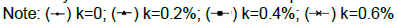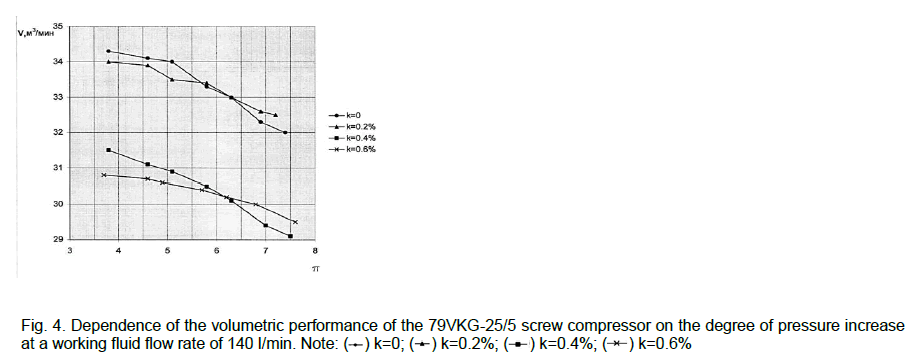Figure 4: Dependence of the volumetric performance of the 79VKG-25/5 screw compressor on the degree of pressure increase at a working fluid flow rate of 140 l/min.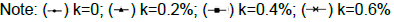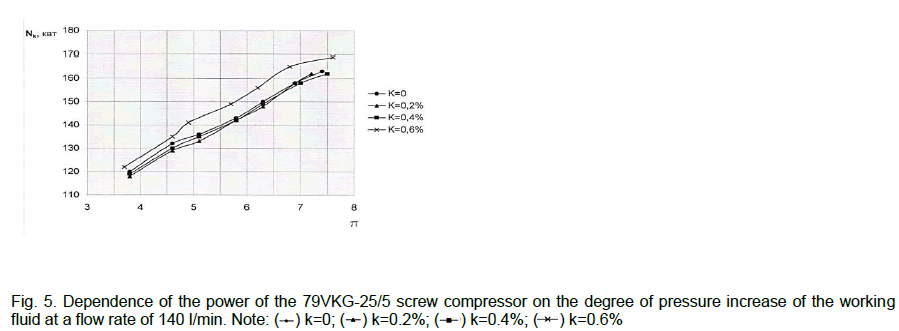Figure 5: Dependence of the power of the 79VKG-25/5 screw compressor on the degree of pressure increase of the working fluid at a flow rate of 140 l/min.Figure 6: Dependence of the isometric efficiency of the 79VKG-25/5 screw compressor on the degree of pressure increase at a working fluid flow rate of 140 l/min.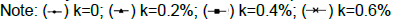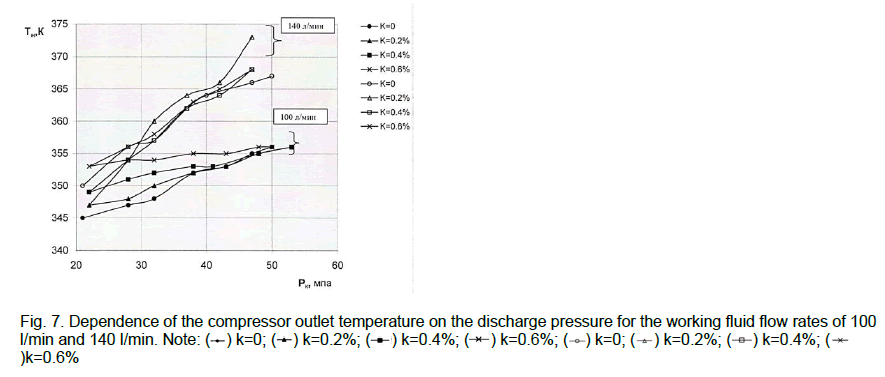Figure 7: Dependence of the compressor outlet temperature on the discharge pressure for the working fluid flow rates of 100 l/min and 140 l/min.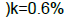The productivity of VKA practically remains constant in the range of changes in the PIB concentration from 0 to 0.2% and decrease on average by 10% at K=0.4 0.6%. The decrease in performance is due to an increase in internal gas flows in the compressor. From the dependences, in the entire range of, the introduction of PIB additives does not lead to a significant increase in the air temperature at the VKA outlet and, therefore, in the pressure in the working cavity of the compressor. Therefore, the main reason for the increase in leakage and a decrease in productivity, apparently, is the deterioration of the sealing properties of the working fluid in the gaps between the housing and the rotors.

## Discussion

The results obtained show the need to continue research in the direction of finding more effective additives for sealing-cooling working fluids of VKA.

The questions about irreversible changes in the properties of dilute solutions, or their degradation, the influence of these changes on the effect of reducing resistance, still remain unclear. There are various reasons as a result of which there are changes in the properties of polymer solutions. The degradation of solutions under the influence of turbulence is of greatest interest in the study of the reduction of resistance.

The action of polymer additives and the degradation of polymer solutions are associated with quantities that determine the flow turbulence, such as the scale of turbulent vortices, the magnitude of velocity pulsations, etc. The latter are determined by the size and flow rate, the viscosity of the liquid, as well as their dimensionless combination-the Reynolds number.

The type of polymer, its molecular weight M, concentration in solution K, temperature and duration of mechanical processing affect the decrease in friction resistance. With an increase in M and K up to a certain value, the effect grows, and a decrease in temperature increases the effect.

In the results of experiments on the study of the rate of degradation are given at a constant rotation rate of the cylinder, but at different temperatures of the solution. The temperature changed in the range from 7 to 38°C.

An increase in the temperature of the solution led to a significant acceleration of degradation. The results obtained did not imply a direct dependence of the degradation rate on temperature. As the temperature changes, the viscosity of the solution changes. It is more likely that it is the change in viscosity that causes a change in the rate of degradation. Some confirmation of this was obtained by studying the influence of the speed of rotation of the cylinders on the rate of degradation. With a decrease in the speed of rotation, the rate of degradation also decreases.

It can be seen that after 40 hours of mechanical stirring of a 0.2% PAA Solution at Re= 5.10^4, a loss of efficiency by 11% was observed. It is noticed that the loss of efficiency is most intense at the beginning of mixing, then it somewhat weakens. The paper presents the results of the effect of polymer additive on hydraulic resistance at high temperature. At Re=const and t=20°C, the relative decrease for an aqueous solution of PAA is 49%; at t=80^0 C, the decrease in the resistance is 42%.

## Conclusion

The results of experimental studies are presented in the form of graphical dependences of the consumed power, volumetric productivity and isothermal efficiency of the 7VKG-25/5 screw compressor on the degree of pressure increase and polyisobutylene concentrations - in the range for the working fluid flow rates V(M1) 100 l/min and V(M2) 140 l/min. From this consideration, it follows that the power consumed by the compressor weakly depends on the PIB concentration in the oil. In the range for all values, there is a tendency to decrease by 10%, which can be explained by a decrease in the power of hydrodynamic losses during the flow of the working fluid in the compressor. When k=0.4% the power consumption increases by 3-5%. The results obtained are in good agreement with the data of the work, in which the optimal concentration of PIB in industrial 45 oil was determined for a centrifugal pump 4K-6, equal to 0.35%.

The productivity of a screw compressor practically remains constant in the range of changes in PIB concentrations from 0 to 0.2% and decreases on average by 10%. The decrease in performance is due to an increase in internal gas flows in the screw compressor. From the presented dependences it follows that in the entire range, the introduction of PIB additives does not lead to a significant increase in the air temperature at the outlet of the screw compressor and, consequently, in the pressure in the working cavity of the compressor. Therefore, the main reason for the increase in leakage and a decrease in productivity, apparently, is the deterioration of the sealing properties of the working fluid in the gaps between the housing and the rotors.